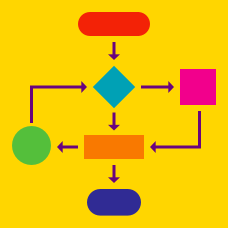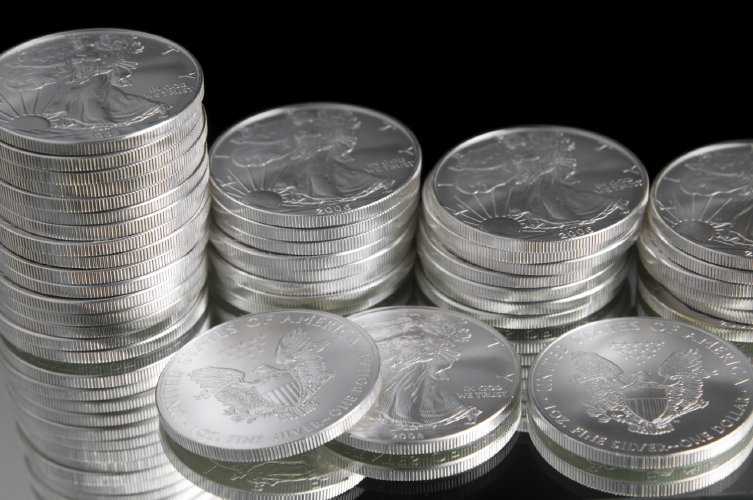Quantitative Finance

# Recursive functionsSuppose we have $2n$ "silver" coins of the same weight. One of them is however fake and it weighs less. In other words we have $2n - 1$ silver coins of equal weight and one fake of lesser weight. We also have a balance scale. We can put any number of coins on each side of the scale at the same time, and the scale will indicate whether the two sides are the same weight or which side is lighter.

Suppose we devise an algorithm that locates the fake coin using the balance scale as few times as possible. What is the maximum number of weighing operations necessary to find the odd coin out?

How many weighing operations would be required for 243 coins if we are as unlucky as possible but we use the optimal algorithm?Consider the task of drawing the markings on a ruler: there is a mark at the halfway point, slightly shorter marks at the quarter intervals and still shorter marks at the eighth intervals. If our desired precision is $\frac{1}{2^n}$ (arbitrary units), we put a mark at every point between $0$ and $2^n$. The middle mark should be $n$ units high the quarter marks should be $n-1$ high, etc. Assuming we have a function mark(x, h) to make a mark $h$ units high at position $x$, the following program does the job of marking a ruler:

 1 2 3 4 5 6 7 8 def rule(left, right, height): if height > 0: x = (left + right) / 2 mark(x, height) rule(left, x, height - 1) rule(x, right, height - 1) else: pass 

Since rule(left, right, height) is recursive we know that it will call itself. Suppose that we call rule(left=0, right=2**10, height=10). During recursive call number $9$ to rule (do not include the initial user call), it will make a mark at position $x$. What is the value of $x$?

The Pascal triangle, shown below, has an interesting property. Every entry is equal to the sum of the two entries above it, except along the left and right edges where the value is always equal to $1$.Write a recursive algorithm that outputs the sum of the numbers on each line. For example the sum of the numbers on the $3$rd line is $1+2+1=4$. What is the sum of the numbers on the $23$rd line?

 1 2 3 4 5 1 define recur(num): 2 if num > 100: 3 return num - 10 4 else: 5 return recur(recur(num + 11)) 

Consider the above recursive algorithm, predict what it will output when $\text{num}=98$.

 1 2 3 4 5 6 7 23 32 62 20 77 42 31 15 14 10 11 48 32 30 14 46 71 31 53 07 82 20 12 78 78 46 24 43 37 16 12 99 15 97 85 13 29 82 71 63 27 75 44 81 80 48 02 45 17 

Consider the square grid above. Suppose a person wants to move from the top left corner towards the bottom right corner. To move, a person can go down or right. Let $S$ be the path traversed such that the sum of the cells in the path is minimized. What is the value of $S$?

×